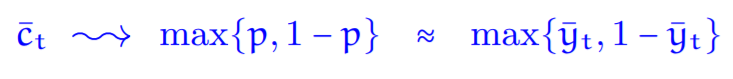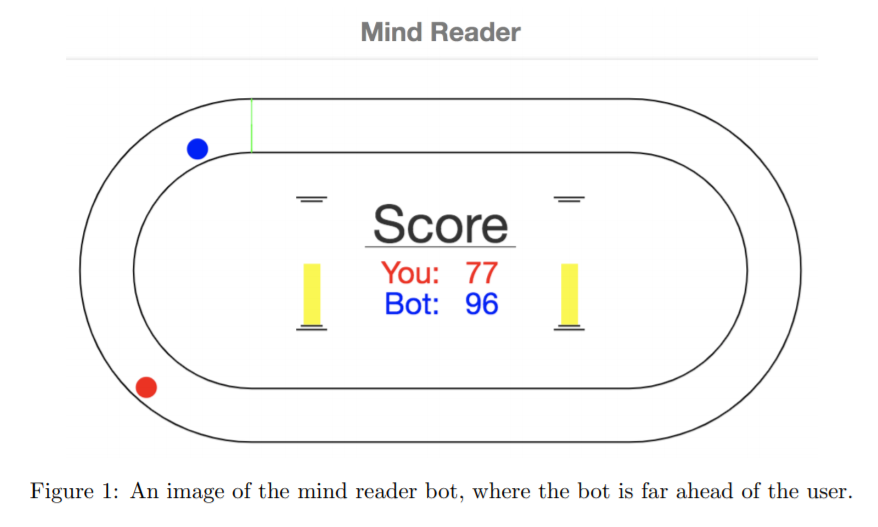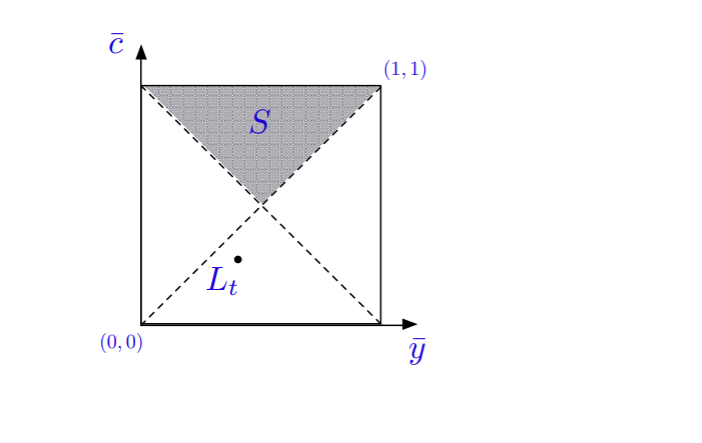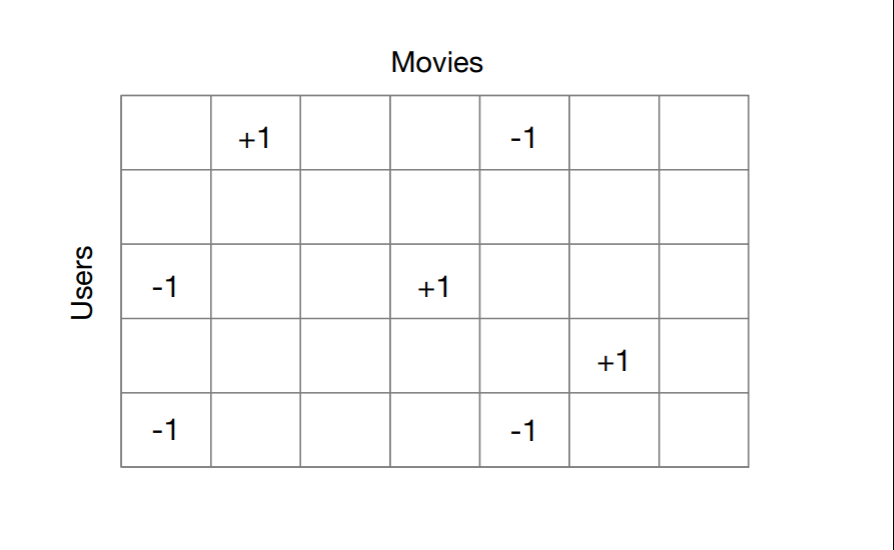# Online Prediction Problem-在线预测算法

## Bit Prediction

### Majority Algorithm• 对于任意的随机二进制序列，都有一个方法，其预测准确性可以和序列中0和1数量不均衡的情况一样好

note： 关于Bit Prediction的majority algorithm，这里有一个小游戏 Mind Reader Bot，游戏规则是：人类选手和计算机比赛赛跑，每次我们选择一个action，向左或是向右，计算机会预测我们的行动，如果预测成功，计算机前进一步；反之我们前行一步。### David Blackwell’s solution：贝叶斯预测

\text { Let } \mathrm{L}_{\mathrm{t}}=\left(\overline{\mathrm{y}}_{\mathrm{t}}, \overline{\mathrm{c}}_{\mathrm{t}}\right)=(\text { prop. of } 1\text { ‘s so far ; prop. of correct so far). }

• \overline{y}_t代表到时刻t，出现1的概率
• \overline{c}_t代表到时刻t，我们预测正确的概率• 上方：c_t>max(\overline{y}_t,1−\overline{y}_t)，我们当前的策略是较好的
• 左方：当前正确率小于1出现的概率, 下一个位置预测0
• 右方：当前正确率小于1出现的概率, 下一个位置预测1
• 下方：我们预测序列下一个值为1的概率是：从点(0.5,0.5)画一条线到L_t，预测1的概率等于直线与y = 0的交点

• 几乎对所有的序列都适用
• 可以很容易证明该方法具有很好的收敛性

## Potential-based Methods

### online supervised learning

movie matrix completion problem. 假设有一个二维矩阵决定一个user何时会喜欢一个电影\sum_{t=1}^{n}\left|\hat{y}_{t}-y_{t}\right| \leq \phi\left(x_{1}, y_{1}, \dots, x_{n}, y_{n}\right)

\sum_{t=1}^{n}\left|\hat{y}_{t}-y_{t}\right| \leq \min _{w \in \mathcal{F}} \sum_{t=1}^{n}\left|\left\langle w, x_{t}\right\rangle- y_{t}\right|+\mathcal{C}_{n}\left(\mathcal{F} ; x_{1}, \ldots, x_{n}\right)

• 第一项用来捕获x/y之间的关系：w是根据历史数据找出的最佳的低秩矩阵，\left\langle w, x_{t}\right\rangle 代表根据wx_t得到预测结果。
• 第二项\mathcal{C}_{n}\left(\mathcal{F} ; x_{1}, \ldots, x_{n}\right)代表得出side information x的复杂度

#### Potential functions.

\hat{y}_{t}=\frac{1}{2}\left(\left(U\left(x_{1}, y_{1}, \ldots, x_{t},+1\right)-U\left(x_{1}, y_{1}, \ldots, x_{t},-1\right)\right)\right.

• U是势函数，我们将取值为1和-1时解的和求平均，即最优预测结果（公式中的减号来源于-1）

• 相比于如SVM这样的学习算法，无需优化求解的过程
• 对于任意长度的数据都适用
• 不依赖于i.i.d假设，更贴近真实世界的数据• d_1是用户数量，d_2是电影数量
• N_c和N_r是出现频率最高的电影和用户

Note: 如果对AI相关的online learning感兴趣，参考 .

### online linear optimization

\sum_{t=1}^{n}\left\langle\hat{y}_{t}, y_{t}\right\rangle \leq \min _{w \in \mathcal{K}} \sum_{t=1}^{n}\left\langle\mathbf{w}, y_{t}\right\rangle+\mathcal{C}_{n}\left(\mathcal{K} ; y_{1}, \ldots, y_{n}\right)
Example. 根据d个专家意见的加权来做预测，其中：

• \overline{y}_t代表d个专家意见的分布；\mathcal{K}是simplex；y_t是loss.

## No-Regret Methods

### No-regret dynamics for zero-sum two-player games

zero-sum two-player games和No-regret dynamics 都是博弈论中的概念：

• zero-sum two-player games：双人零和博弈，又称零和游戏，与非零和博弈相对，是博弈论的一个概念，属非合作博弈。它是指参与博弈的各方，在严格竞争下，一方的收益必然意味着另一方的损失，博弈各方的收益和损失相加总和永远为“零”，双方不存在合作的可能。
• No-regret dynamics：是指在知道对手策略的情况下，博弈者也不会有动力改变当前策略，即达到了纳什均衡 

Note: 对于零和而言，在某个状态下计算所有可能的action时，对于某一方，总有一系列后续的应对策略，能够达到最终胜利（或者至少平局）；也就是有所谓的“必胜策略”。这一点一开始会让人觉得有点反直觉：是不是某些状态下，我们无法确认能够有必胜策略呢？答案是否定的。如果我们并不能找到这样一个必胜策略，就意味着无论如何选择action，对手总有办法阻止你必胜，由于游戏是零和的，这也意味着对手有必胜策略 

• minimax是一种悲观算法，即假设对手每一步都会将我方引入从当前看理论上价值最小的格局方向，即对手具有完美决策能力。因此我方的策略应该是选择那些对方所能达到的让我方最差情况中最好的，也就是让对方在完美决策下所对我造成的损失最小。
• Minimax不找理论最优解，因为理论最优解往往依赖于对手是否足够愚蠢，Minimax中我方完全掌握主动，如果对方每一步决策都是完美的，则我方可以达到预计的最小损失格局，如果对方没有走出完美决策，则我方可能达到比预计的最悲观情况更好的结局。总之我方就是要在最坏情况中选择最好的。

• M_{i, j} (d_1 \times d_2的矩阵) 代表player 1: 在自己选择action i，player 2选择action j时的代价
• -M_{i, j}代表player 2的代价

\min _{q \in \Delta_{d_{1}}} \max _{j \in\left[d_{2}\right]} q^{T} M e_{j}=\max _{p \in \Delta_{d_{2}}} \min _{i \in\left[d_{1}\right]} \frac{T}{i} M p=\min _{q \in \Delta_{d_{1}}} \max _{p \in \Delta_{d_{2}}} q^{T} M p

\frac{1}{n} \sum_{t=1}^{n} q_{t}^{\top} M p_{t}-\min _{q \in \Delta_{d}} \frac{1}{n} \sum_{t=1}^{n} q^{\top} M p_{t} \leq \frac{1}{n} \operatorname{Reg}_{n}^{(1)}

\frac{1}{n} \sum_{t=1}^{n}\left(-q_{t}^{\top} M\right) p_{t}-\min _{p \in \Delta_{d_{2}}} \frac{1}{n} \sum_{t=1}^{n}\left(-q_{t}^{\top} M\right) p \leq \frac{1}{n} \operatorname{Reg}_{n}^{(2)}

\max _{\mathbf{p} \in \Delta_{\mathrm{d}_{2}}} \overline{\mathbf{q}}^{\top} M p-\min _{\mathbf{q} \in \Delta_{\mathrm{d}_{1}}} \mathbf{q}^{\top} M \overline{\mathbf{p}} \leq \frac{1}{n}\left(\operatorname{Reg}_{n}^{(1)}+\operatorname{Reg}_{n}^{(2)}\right)

\min _{q \in \Delta_{d_{1}}} q^{T} M \bar{p} \leq \max _{p \in \Delta_{d_{2}}} \min _{q \in \Delta_{d_{1}}} q^{T} M p=\min _{p \in \Delta_{d_{1}}} \max _{p \in \Delta_{d_{2}}} q^{T} M p \leq \max _{p \in \Delta_{d_{2}}} \bar{q}^{T} M p

\sqrt{\sum_{t=1}^{n}\left|y_{t}-\mathcal{M}_{t}\right|^{2}}

### Optimistic Mirror Descent

Optimistic Mirror Descent是优化的update method，具体过程：
\begin{aligned} g_{t+1} &=\operatorname{argmin}_{v \in \mathcal{K}} \eta\left\langle v, y_{t}\right\rangle+ D_{\phi}\left(v, g_{t}\right) \\ \hat{y}_{t+1}=& \operatorname{argmin}_{v \in \mathcal{K}} \eta\left\langle v, M_{t+1}\right\rangle+ D_{\phi}\left(v, g_{t+1}\right) \end{aligned}

Optimistic Mirror Descent的一些相关应用  ：

• can be related to Mirror Prox of Nemirovskii when applied to structured optimization (saddle point optimization)
• results extend to partial information settings
• application to smooth convex programming and MaxFlow
• recent applications to GAN training